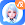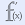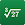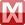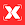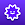# Cymath - Math Problem Solver

by Cymath LLC
5,000,000+ installs

Android application Cymath - Math Problem Solver developed by is listed under category Education. According to Google Play Cymath - Math Problem Solver achieved more than 5,000,000 installs. Cymath - Math Problem Solver currently has 70,082 ratings with average rating value of 4.45. The current percentage of ratings achieved in last 30 days is 0.81%, percentage of ratings achieved in last 60 days is 1.27%. Cymath - Math Problem Solver has the current market position #12452 by number of ratings. A sample of the market history data for Cymath - Math Problem Solver can be found below. Last update on 2023-12-04.

Title: Cymath - Math Problem Solver Cymath LLC Education Free Android
Total ratings: 70082 0.81% 1.27% 4.45
Installs (achieved): 5,000,000+ 6,130,657
5 star ratings: 54,967 6,010 1,423 869 6,801

2023-01-15: Android application Cymath - Math Problem Solver achieved 5,000,000 installs. Android application Cymath - Math Problem Solver achieved 1,000,000 installs. Android application Cymath - Math Problem Solver achieved 500,000 installs. Android application Cymath - Math Problem Solver achieved 100,000 installs. Android application Cymath - Math Problem Solver achieved 50,000 installs.

Total number of ratings
Total number of active users rated for Cymath - Math Problem Solver.

Total number of installs (*estimated)
Estimation of total number of installs on Google Play. Approximated from number of ratings and install bounds achieved on Google Play.

Average rating
Average rating value on Google Play. Given by active users of the application.